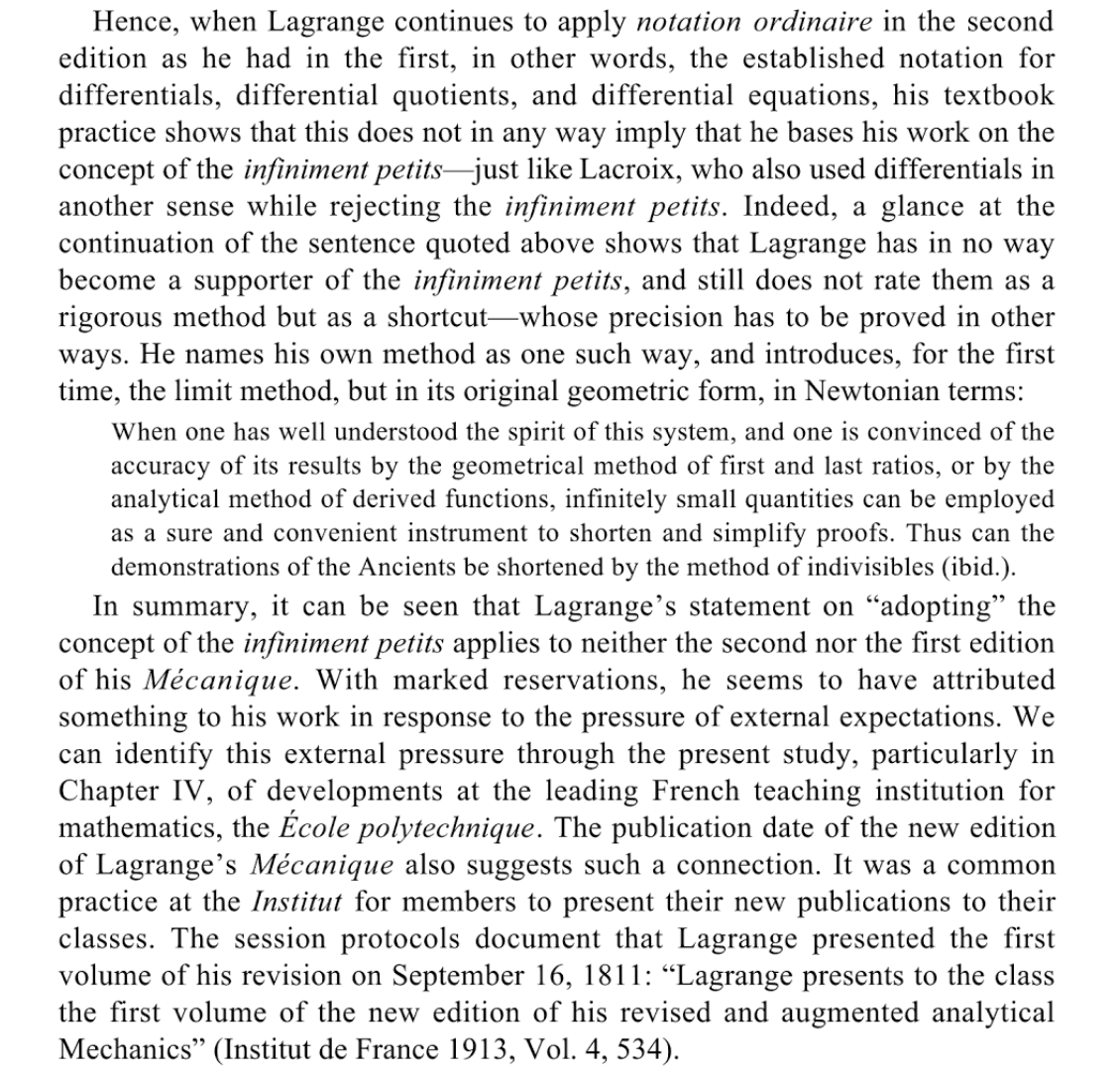# Did Lagrange change his mind about infinitesimals?

Lagrange is famous for his attempt to found analysis algebraically using power series expansions, an approach that, as we know today, is limited to analytic functions. Lagrange is also known as the initiator of a contest at the French Academy at the end of the 18th century, for the best essay on justifying infinity and infinitesimals. L. Carnot did not win the contest but his essay eventually became very popular. The outcome of the contest was something of a disappointment to its organizers who did not find any of the submissions fully satisfactory.

What is less known is that toward the end of his career, and already in the 19th century, Lagrange fully embraced infinitesimals in the introduction to the second, 1811 edition of his book Analytical Mechanics, in the following terms:

Once one has duly captured the spirit of this system [i.e., infinitesimal calculus], and has convinced oneself of the correctness of its results by means of the geometric method of the prime and ultimate ratios, or by means of the analytic method of derivatives, one can then exploit the infinitely small as a reliable and convenient tool so as to shorten and simplify proofs. (translation mine)

Is there any work analyzing the actual use of infinitesimals by Lagrange in the body of the 1811 edition of his book?

I just noticed that in the article

Carpinteri, Alberto; Paggi, Marco. Lagrange and his Mécanique analytique: from Kantian noumenon to present applications. Meccanica 49 (2014), no. 1, 1-11

the authors comment as follows on the second edition:

In the new formulation of mechanics proposed by Lagrange, the quantity of primary importance is no longer the force, but the work done by forces for infinitesimal arbitrary movements. (page 6)

The authors then go on to quote a passage from Lagrance:

Lagrange thus arrived at the formulation of the following expression ([11, 12], p. 21): "the principle of virtual velocities can be considered as very general by expressing it in the following manner: if any system, composed of any number of points or bodies, each of which solicited by any force, is in equilibrium; and if this system is given any small movement, in virtue of which each point travels by an infinitely small displacement, which shall express its virtual velocity - then the sum of the forces, each one multiplied by the displacement travelled (in its direction) from its point of application, shall always be equal to zero; considering the displacements travelled in the same direction of the forces as positive and small; and the travelled displacements in opposite direction as negative". (emphasis added)

• I don't feel very strongly about but since Lagrange did not know nonstandard analysis or modern logic, I am not sure these additional tags are appropriate. Apr 26, 2017 at 10:45
• I would remove the tags for logic and nonstandard analysis. That is a particular approach to infinitesimals, one way of presenting rigorous ideas like Lagrange's, but it's not the only approach, and it is distinct from the historical question here.
– user44143
Apr 26, 2017 at 10:45
• Fine, @MattF... Apr 26, 2017 at 10:46